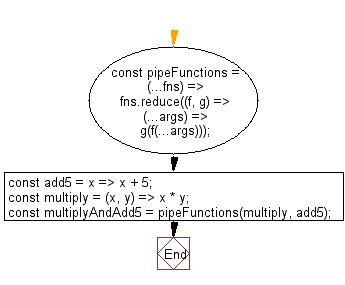# JavaScript: Perform left-to-right function composition

## JavaScript fundamental (ES6 Syntax): Exercise-165 with Solution

Write a JavaScript program to perform left-to-right function composition.

• Use Array.prototype.reduce() with the spread operator (...) to perform left-to-right function composition.
• The first (leftmost) function can accept one or more arguments; the remaining functions must be unary.

Sample Solution:

JavaScript Code:

``````//#Source https://bit.ly/2neWfJ2
const pipeFunctions = (...fns) => fns.reduce((f, g) => (...args) => g(f(...args)));
const add5 = x => x + 5;
const multiply = (x, y) => x * y;

```
```

Sample Output:

```15
37
```

Pictorial Presentation:Flowchart:Live Demo:

See the Pen javascript-basic-exercise-165-1 by w3resource (@w3resource) on CodePen.

Improve this sample solution and post your code through Disqus

What is the difficulty level of this exercise?

Test your Programming skills with w3resource's quiz.

﻿